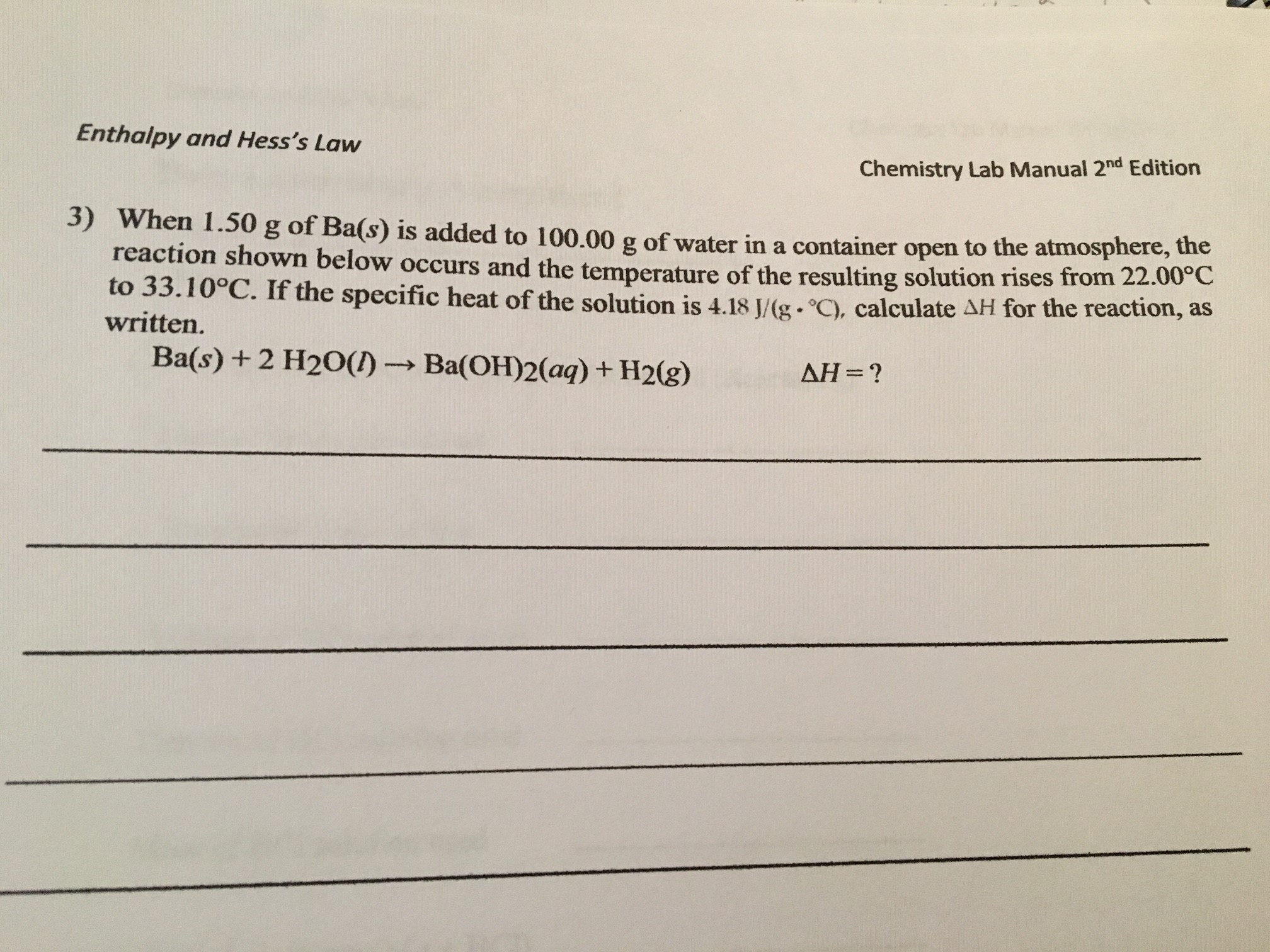# Enthalpy and Hess's Law Chemistry Lab Manual 2nd Edition 3) When 1.50 g of Ba(s) is added to 100.00 g of water in a container open to the atmosphere, the reaction shown below occurs and the temperature of the resulting solution rises from 22.00C to 33.10°C. If the specific heat of the solution is 4.18 J/(g. °C) , calculate AH for the reaction, as written. Ba(s) + 2 H2O() AH ? Ba(OH)2(aq) + H2(g)

Question

thank you so much! :)help_outlineImage TranscriptioncloseEnthalpy and Hess's Law Chemistry Lab Manual 2nd Edition 3) When 1.50 g of Ba(s) is added to 100.00 g of water in a container open to the atmosphere, the reaction shown below occurs and the temperature of the resulting solution rises from 22.00C to 33.10°C. If the specific heat of the solution is 4.18 J/(g. °C) , calculate AH for the reaction, as written. Ba(s) + 2 H2O() AH ? Ba(OH)2(aq) + H2(g) fullscreen

### Want to see this answer and more?

Experts are waiting 24/7 to provide step-by-step solutions in as fast as 30 minutes!*